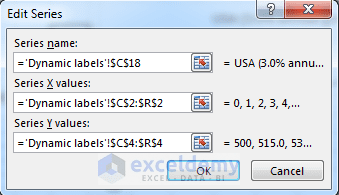Disclosure: This post may contain affiliate links, meaning when you click the links and make a purchase, we receive a commission.

# Excel Charts with Dynamic Title and Legend Labels

Dynamism is everywhere!

Then why not with Excel?

Creating dynamic charts in Excel is possible in many ways.

See the dynamic image below. Like it? If the image was static, would you like it in the same way?

Observe the above image carefully!

When I am changing the values of cells C4, C5, C7 or C8, the chart is showing different Titles and Legends.

Now let’s take a look at the chart more deeply! Below is a static image of the worksheet for your close observation (so, static things have value too!).

The worksheet is showing the GDP forecasting of US and China. You will see this type of forecasting in practical life.

• GDP for US is set 500 (see cell C4) with a growth rate of 3% (cell C5).
• GDP for China is set 250 (see cell C7) with a growth rate of 10% (cell C8).

This forecasting wants to know what will be the scenario if the above GDP and growth rate continue for the next 15 years? That we want to see with a chart.

Read More: Sparklines in Excel (Insert, Change, & Modify to Show Data Trends)!

## Introduction to worksheet data and formulas

Let me introduce you with the worksheet data and formulas used in the calculations.

1. From cell C2 to R2, values from 0 to 15 are set. You can think these values as years. 0 is the starting point (year) and 1 is regarded as after one year.
2. Check out the cell D5. It holds this formula: =C5. So it just copies of the value of cell C5 and it is 3%. Then I have just copied this formula for other cells on the right (up to cell R5). So all the cells are holding the value 3% in them.
3. Check out the formula in cell D4. It is: =C4*(1+D5). The values of C4 and D5 are 500 and 3% respectively. And the result is 515. I have copied this formula for other cells on the right (up to cell R4).
4. In the above-mentioned ways, I have also made formulas for ranges D8: R8 and D7: R7.
5. Now take a look the formula in cell D10: =IF(D7>=D4,1,0). If the value of cell D7 is greater than or equal to the value of cell D4, it will return 1, otherwise 0. I copied this formula for other cells on the right (up to cell R10). First time 1 is returned in cell N10. The value of N2 is 11. So after 11 years China GDP (with 713.3) has crossed the GDP of US (with 692.1).
6. Check the formula in cell, C14: =IFERROR (MATCH (1, D10: R10, 0),”none”). Look at the MATCH function part. It searches the value 1 in cell range D10: R10, if it finds any, returns the position of 1 in the range. If it does not find any, returns an error. IFERROR function catches that error, and returns “none”.
7. Now see the formula in cell C17: =IF (C14=”none”, “US stays on top”, “Chinese GDP to pass US GDP in Year “&TEXT(C14,”0”)). If C14 is none (it means there is no 1 in the range D10: R10), the IF function returns “US stays on top”, otherwise IF function returns “Chinese GDP to pass US GDP in Year “&TEXT(C14,”0”)”. TEXT function returns the value of cell C14 in General format.
8. Other two formulas are in cells C18 and C19. C18: =”USA (“&TEXT(C5,”0.0%”) &” annual growth)” and C19: =“China (“&TEXT(C8,”0.0%”) &” annual growth)”. These two formulas are self-explanatory.

Let’s check the whole worksheet with a new value in cell C8 (input value 7%). And now look at the following image.

No value in the range D10: R10 shows 1. So the cell C14 shows “none”.

And the Title is showing as “US stays on top”.

The chart also shows the reflection of the changes (Title, Legend labels).

Want me to guide you on Advanced Excel Charting?

Join Leila Gharani’s

Excel Charts: Visualization Secrets for Impressive Charts course!

[I personally guarantee that this course will make you the Data Visualization Star at your company!]

## Let’s create the chart with dynamic title and labels

Creating the chart is really simple.

1. Select an empty cell in the worksheet and create an XY chart (scatter with smooth lines).
2. Open the Select Data Source dialog box (Chart Tools (Design) -> Data -> Select Data).
4. In the Edit Series dialog box, as Series name, point to cell reference C18, as Series X values, point to cell range C2: R2 and as Series Y values, point to range C4: R4. When done, click OK.Input values in Edit series dialog box.

5. Again open the Edit Series dialog box clicking on the Add button. This time as Series name, point to cell reference C19, as Series X values, point to cell range C2: R2 and as Series Y values, point to range C7: R7. When done, click OK.
6. Close the Select Data Source dialog box. You will get the chart.

So, you’re done. Now play with the chart.